Next: Vector Area Up: Vectors and Vector Fields Previous: Coordinate Transformations

# Scalar Product

A scalar quantity is invariant under all possible rotational transformations. The individual components of a vector are not scalars because they change under transformation. Can we form a scalar out of some combination of the components of one, or more, vectors? Suppose that we were to define the percent'' product,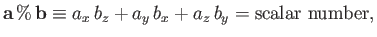(A.23)

for general vectors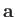and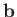. Is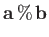invariant under transformation, as must be the case if it is a scalar number? Let us consider an example. Suppose that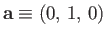and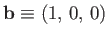. It is easily seen that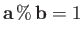. Let us now rotate the coordinate axes throughabout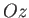. In the new coordinate system,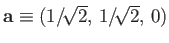and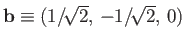, giving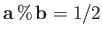. Clearly,is not invariant under rotational transformation, so the previous definition is a bad one.

Consider, now, the dot product or scalar product:(A.24)

Let us rotate the coordinate axes though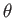degrees about. According to Equations (A.20)-(A.22),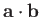takes the form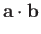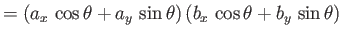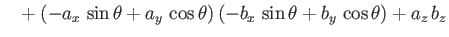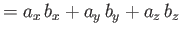(A.25)

in the new coordinate system. Thus,is invariant under rotation about. It is easily demonstrated that it is also invariant under rotation about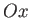and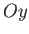. We conclude thatis a true scalar, and that the definition (A.24) is a good one. Incidentally,is the only simple combination of the components of two vectors that transforms like a scalar. It is readily shown that the dot product is commutative and distributive: that is,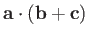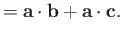(A.26)

The associative property is meaningless for the dot product, because we cannot have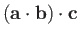, asis scalar.

We have shown that the dot productis coordinate independent. But what is the geometric significance of this property? In the special case where, we get(A.27)

So, the invariance of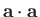is equivalent to the invariance of the magnitude of vectorunder transformation.

Let us now investigate the general case. The length squared of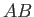in the vector triangle shown in Figure A.6 is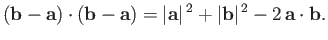(A.28)

However, according to the cosine rule'' of trigonometry,(A.29)

wheredenotes the length of side. It follows that(A.30)

In this case, the invariance ofunder transformation is equivalent to the invariance of the angle subtended between the two vectors. Note that if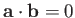then either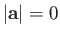,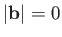, or the vectorsandare mutually perpendicular. The angle subtended between two vectors can easily be obtained from the dot product: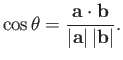(A.31)The workperformed by a constant forcethat moves an object through a displacementis the product of the magnitude oftimes the displacement in the direction of. If the angle subtended betweenandisthen(A.32)

The workperformed by a non-constant force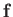that moves an object through an infinitesimal displacement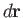in a time interval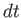is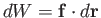. Thus, the rate at which the force does work on the object, which is usually referred to as the power, is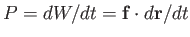, or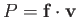, whereis the object's instantaneous velocity.Next: Vector Area Up: Vectors and Vector Fields Previous: Coordinate Transformations
Richard Fitzpatrick 2016-03-31